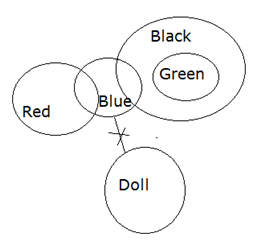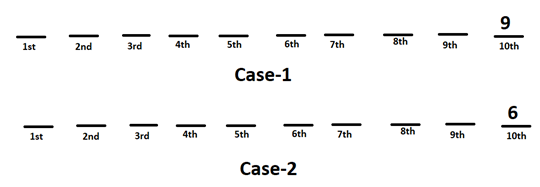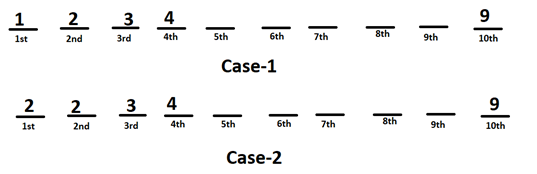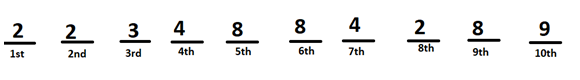# RRB Clerk Mains Reasoning Ability Questions 2021 (Day-01)

Dear Aspirants, Our IBPS Guide team is providing new series of Reasoning Questions for RRB Clerk Mains 2020 so the aspirants can practice it on a daily basis. These questions are framed by our skilled experts after understanding your needs thoroughly. Aspirants can practice these new series questions daily to familiarize with the exact exam pattern and make your preparation effective.

Start Quiz

Order and ranking

Directions (1-2): Study the following information carefully and answer the questions given below:

Eight persons R, V, M, K, T, G, S and P are arranged according to their weight. Each one of them likes different colour White, Orange, Blue, Green, Grey, Brown, Yellow and Black.

Only two persons are heavier than V. G is heavier than T but lighter than S. R likes Blue colour and is lighter than M. T is not the lightest. R is heavier than S. The one who is the lightest likes yellow colour. K likes Grey colour. K is heavier than G but lighter than S. The one who likes Black is heavier than Green but lighter than the one who likes white. The one who likes Brown is heavier than the one who likes orange but lighter than the one who likes Green.

1) How many persons are heavier than K?

A.4

B.5

C.6

D.3

E. None of these

2) Which of the following person likes Brown?

A.T

B.G

C.S

D.P

E. None of these

Syllogism

Direction (3): In each of the questions below are given three statements, followed by conclusions: I, II and III. You have to take the given statements to be true even if they seem to be at variance from commonly known facts. Read the conclusions and then decide which of the given conclusions logically follows from the given statements disregarding commonly known facts.

3) Statements:

Some red are blue.

No blue is a doll.

Only a few blue are black.

All green is black.

Conclusions:

I). Some green are blue.

II). Some black are not doll.

III). All red can be doll.

IV). No green is a doll.

A. Only IV follows

B. Only II follows

C. Only I and IV follow

D. Only I and II follow

E. None follows

Inequality

Directions (4-5): In these questions, relationship between different elements is shown in the statements. These statements are followed by two conclusions:

4) Statements:

T ≥ H > K = G ≥ S ;   D ≤ W< K < F ≤ P ;   R > F ≤ M

Conclusions:

I).H > P

II). M ≥ K

III). D < H

A. All follow

B. Only I and II follow

C. Only I follow

D. Only III follows

E. None of these

5) Statements:

H > P ≥ K ≥ L ;   P ≤ Q < E ≥ U ;   S ≥ E ≤ V

Conclusions:

I). E > U

II). L ≥ K

III).P ≤ S

A. None follows

B. Only I and II follow

C. Only III follows

D. Only I and III follow

E. None of these

Puzzle

Directions (6-8): Refer to the data below and answer the questions that follow.

Ajay went to a bank to deposit a sum of money in his account. The banker asked for the account number from Ajay and following points are known about his account number.

Note: The account number is of 10 digits and does not start with zero. Zero is not considered as even number.

1)  The last digit of the account number is divisible by 3 but is not 3.

2) The difference of first and last digit is greater than 6.

3) The second digit of the account number is an even prime number.

4) The 2nd, 3rd and 4th numbers are consecutive numbers starting from 2nd digit.

5) The 5th and 6th digit are even numbers greater than 4

6) The sum of 6th and 1st digit is greater than 9.

7) The 8th digit is same as the 1st digit and 7th digit is sum of 1st and 2nd digit.

8) The 9th and 5th digits are same and are more than 7.

A.3

B.4

C.8

D.6

E.2

A.13

B.14

C.18

D.16

E.12

#### 8) How many even digits are there in Ajay’s Account number?

A.6

B.8

C.7

D.5

E.9

Alphabet series

Direction (9-10): The questions are based on the following arrangement. Study the arrangement carefully to answer these questions.

2837 SIMPLE   6784 CHASE   2658 BYTES   1753 BLEND

9) If ‘1’ is added to each odd digit and “2” is subtracted from each even digit of every number then, how many such newly formed 4-digit numbers are there, which are having three or more even numbers?

A.1

B.2

C.3

D.4

E.None

10) If the words are arranged in alphabetical order along with the preceding number then digits within the number are arranged in ascending order then which word formed with biggest number?

A.SIMPLE

B.CHASE

C.BLEND

D.BYTES

E.None of these

Directions (1-2) :

M(White) > R(Blue) > V(Black) > S(Green) > K(Grey) > G(Brown) > T(Orange) > P (yellow)H > K < F ≤ P —–> (H > P is false)

M ≥ F > K ——> (M ≥ K is false)

D ≤ W < K < H —–> (D < H is true)

Only conclusion III follows. Thus, d is the correct answer.

E ≥ U — -> (E > U is false)

L ≤ K ——> (L ≥ K is false)

P ≤   Q < E ≤   S —–> (P ≤ S is false)

None follows. Thus, a is the correct answer.

Directions (6-8) :

1) The last digit of the account number is divisible by 3 but the digit is not 3.

That means the digit will be 6 or 9.2) The difference of first and last digit is greater than 6.

That means the last digit is 9 and 1st digit is 1 or 2.

3) The second digit of the account number is an even prime number.

4) The 2nd, 3rd and 4th numbers are consecutive numbers starting from 2nd digit.5) The 5th and 6th digit are even numbers greater than 4

It means both 5th and 6th digit are either 6 or 8.

6) The sum of 6th and 1st digit is greater than 9.

That means the 1st digit is 2and 6th digit is 8.

7) The 8th digit is same as the 1st digit and 7th digit is sum of 1st and 2nd digit.

Hence 8th digit is 2 and 7th digit is 4.

8) The 9th and 5th digits are same and are more than 7.

It means 9th and 5th digits are 8 (ref point (5))Hence Ajay account number is 2234884289

2837 SIMPLE   6784 CHASE   2658 BYTES   1753 BLEND

0648 SIMPLE    4862 CHASE   0466 BYTES   2864 BLEND